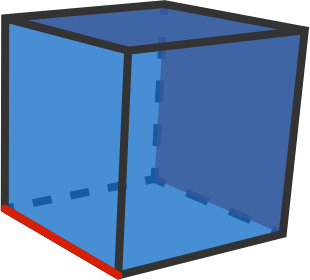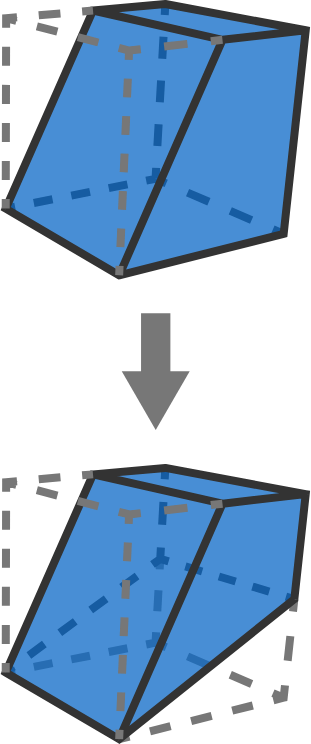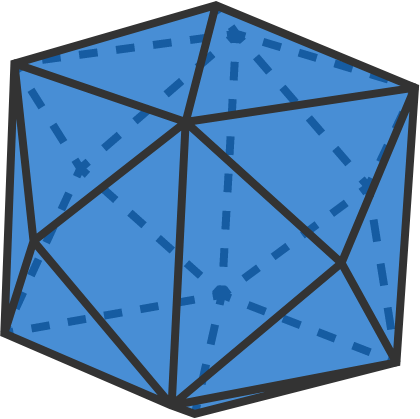# Chopping Up the Cube

Geometry Level 3

The lower left edge of a cube has two opposite faces (the top and rightmost) with which it doesn't share a vertex. This is also true for the other 11 edges.We first cut off a wedge with a plane going through the bottom left edge and the line segment dividing the top face into two equal halves. We do the same for the other opposite face at right.Since the cube has 12 edges, we need a total of $2\times 12 =24$ straight cuts as described above to come up with the polyhedron shown below. If the volume of this core polyhedron is $V_P,$ and the volume of the original cube is $V,$ then $V_P = \frac{1}{n} V.$

What is $n?$×